## You attach a meter stick to an oak tree, such that the top of the meter stick is 1.471.47 meters above the ground. Later, an acorn falls fro

Question

You attach a meter stick to an oak tree, such that the top of the meter stick is 1.471.47 meters above the ground. Later, an acorn falls from somewhere higher up in the tree. If the acorn takes 0.2810.281 seconds to pass the length of the meter stick, how high h0h0 above the ground was the acorn before it fell, assuming that the acorn did not run into any branches or leaves on the way down?

in progress 0
2 months 2021-07-25T16:40:52+00:00 1 Answers 6 views 0

The value is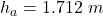Explanation:

From the question we are told that

The  height of the top meter stick above the ground is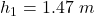The  time taken for the acorn to pass the length of the  stick is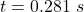Generally the height of the acorn at the point it is the same height with the metered stick is mathematically represented as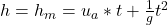Here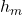is height of the meter stick and the value is 1 m (This because we are told in the question that the stick is 1 meter in length ( a meter stick))

So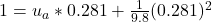=>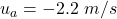Generally the velocity of the acorn just before passing the top of the meter stick is mathematically represented by a kinematic equation as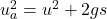here  u is zero since the acorn started from rest

So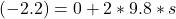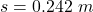Generally the height of the acorn is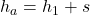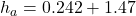\